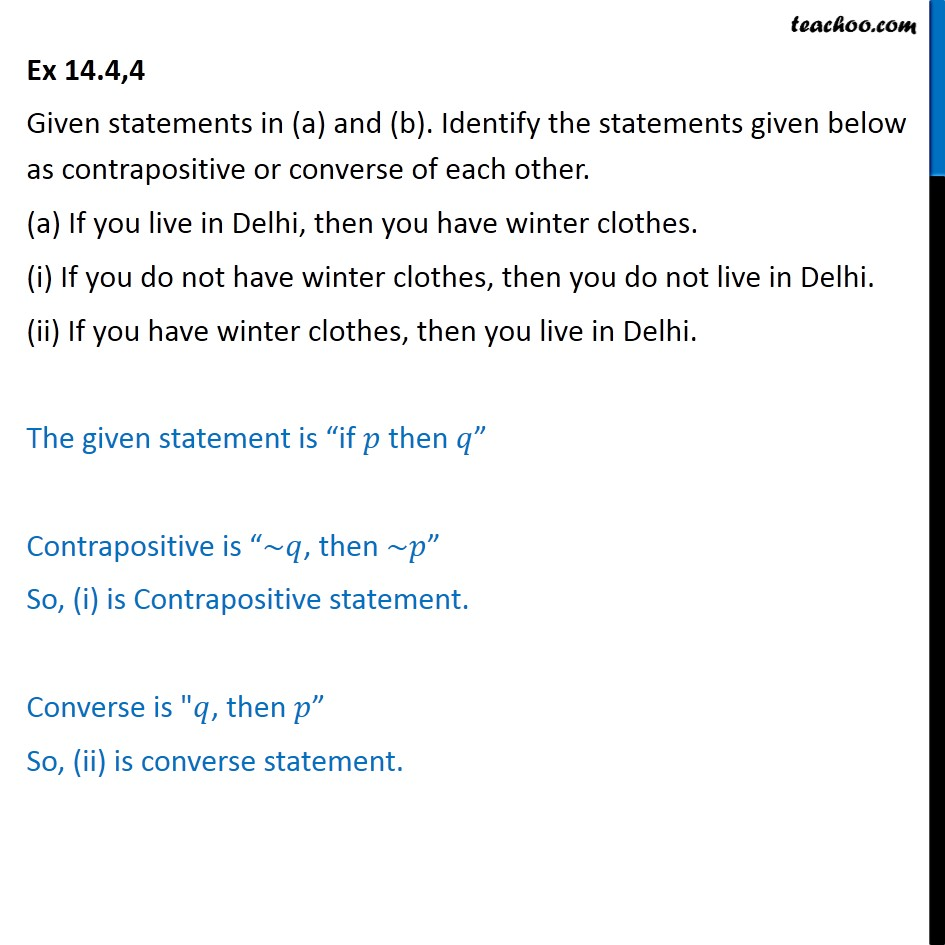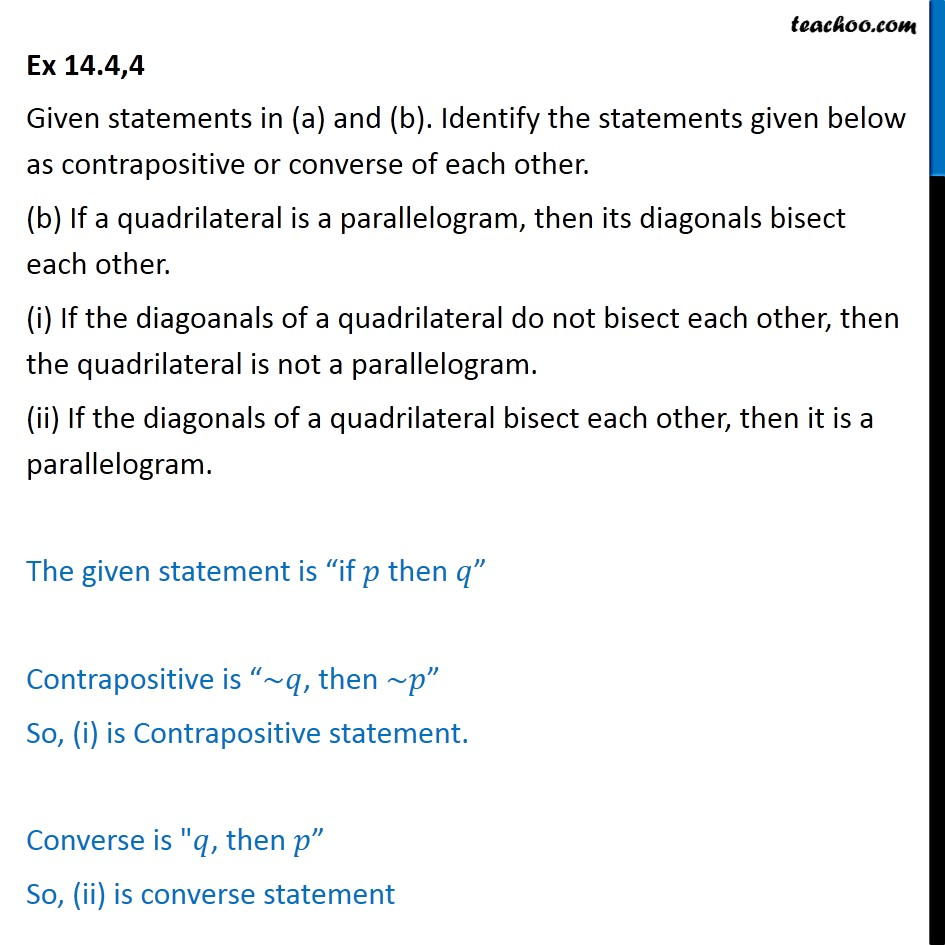Subscribe to our Youtube Channel - https://you.tube/teachoo

1. Chapter 14 Class 11 Mathematical Reasoning
2. Serial order wise
3. Ex 14.4

Transcript

Ex 14.4,4 Given statements in (a) and (b). Identify the statements given below as contrapositive or converse of each other. (a) If you live in Delhi, then you have winter clothes. (i) If you do not have winter clothes, then you do not live in Delhi. (ii) If you have winter clothes, then you live in Delhi. The given statement is “if 𝑝 then 𝑞” Contrapositive is “~𝑞, then ~𝑝” So, (i) is Contrapositive statement. Converse is "𝑞, then 𝑝” So, (ii) is converse statement. Ex 14.4,4 Given statements in (a) and (b). Identify the statements given below as contrapositive or converse of each other. (b) If a quadrilateral is a parallelogram, then its diagonals bisect each other. (i) If the diagoanals of a quadrilateral do not bisect each other, then the quadrilateral is not a parallelogram. (ii) If the diagonals of a quadrilateral bisect each other, then it is a parallelogram. The given statement is “if 𝑝 then 𝑞” Contrapositive is “~𝑞, then ~𝑝” So, (i) is Contrapositive statement. Converse is "𝑞, then 𝑝” So, (ii) is converse statement

Ex 14.4